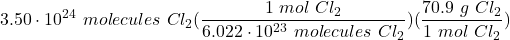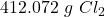## If I had 3.50 x 10 24molecules of Cl2 gas, how many grams is this?

Question

If I had 3.50 x 10 24molecules of Cl2 gas, how many grams is this?

in progress 0
3 weeks 2021-08-23T23:42:57+00:00 1 Answers 1 views 0

412 g Cl₂

General Formulas and Concepts:

Atomic Structure

• Moles
• Avogadro’s Number – 6.022 × 10²³ atoms, molecules, formula units, etc.

Stoichiometry

• Using Dimensional Analysis

Explanation:

Step 1: Define

[Given] 3.50 × 10²⁴ molecules Cl₂

[Solve] grams Cl₂

Step 2: Identify Conversions

[PT] Molar Mass of Cl – 35.45 g/mol

Molar Mass of Cl₂ – 2(35.45) = 70.9 g/mol

Step 3: Convert

1. [DA] Set up:2. [DA] Divide/Multiply [Cancel out units]:Step 4: Check

Follow sig fig rules and round. We are given 3 sig figs.

412.072 g Cl₂ ≈ 412 g Cl₂